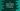Let’s write a program that keeps reading user inputs until it receive -1 as input. You can say that -1 will exit the program. These types of programs are used in many scenarios, e.g. if you have a program that runs for infinite time, you can add an exit point for a special input.

We will also find the total count of positive and negative values the user has entered before -1.

### Algorithm:

The idea is to write an infinite loop that will break only if the user enters -1. while loop is best to write an infinite loop.

• Run an infinite while loop
• Keep reading the user input
• If user enters -1, exit from the loop.

Let’s write it down in code:

### Method 1: By using break:

By using the break statement, we can exit from a loop. To run a while loop for infinite time, we need to pass 1 to its condition. 1 is considered as true and it will keep the loop running for infinite time.

``````#include <stdio.h>

int main()
{
int number;

printf("Enter -1 to exit from the loop:\n");

while (1)
{
printf("Enter a number: ");
scanf("%d", &number);

if (number == -1)
{
break;
}
}
return 0;
}``````

Here,

• number is an integer value to hold the user input number.
• The while loop runs for infinite time.

• On each iteration, it is asking the user to enter a number. It reads that number and stores it in the number variable.
• If the value of number is -1 or if user has entered -1 it exits from the loop by using break.

If you run this program, it will give output as like below:

``````Enter -1 to exit from the loop:
Enter a number: 1
Enter a number: 3
Enter a number: 4
Enter a number: 1019
Enter a number: 10
Enter a number: -1``````

### Method 2: Without using break:

Another way is to do this by assigning a initial value to number. If we assign an initial value to number, we can add a check to the value of number in the while loop.

``````#include <stdio.h>

int main()
{
int number = 0;

printf("Enter -1 to exit from the loop:\n");

while (number != -1)
{
printf("Enter a number: ");
scanf("%d", &number);
}
return 0;
}``````

For this program, the while loop will keep running till the value of number is not equal to -1. It will stop if it is -1.

It will work in a similar way:

``````Enter -1 to exit from the loop:
Enter a number: 1
Enter a number: 19
Enter a number: 199
Enter a number: -998
Enter a number: -1``````

## Method 3: By using a do-while loop:

We can also use a do-while loop:

``````#include <stdio.h>

int main()
{
int number = 0;

printf("Enter -1 to exit from the loop:\n");

do
{
printf("Enter a number: ");
scanf("%d", &number);
} while (number != -1);

return 0;
}``````

It is similar to the above program. The only difference is that the condition is checked in the while statement and the code is written in the do block.

It will give similar output.

``````Enter -1 to exit from the loop:
Enter a number: 0099
Enter a number: -1234
Enter a number: 939393
Enter a number: -1``````

## Method 4: Find the count of positive and negative numbers:

We can also count the positive and negative numbers. We need to defined two variables to hold the count and initialize them as 0. Inside the loop, based on the user input, we will increment any of these variables.

``````#include <stdio.h>

int main()
{
int number = 0, positive = 0, negative = 0;

printf("Enter -1 to exit from the loop:\n");

do
{
printf("Enter a number: ");
scanf("%d", &number);

if (number >= 0)
{
positive++;
}
else
{
negative++;
}

} while (number != -1);

printf("Total positive numbers: %d, negative numbers: %d\n", positive, negative);

return 0;
}``````

Here,

• positive and negative are two variables to hold the positive and negative numbers count.
• Inside the loop, if number is greater than or equal to 0, positive is incremented by 1. Else, negative is incremented by 1.
• After the loop ends, it prints the total positive and negative numbers count.

It will give output as like below:

``````Enter -1 to exit from the loop:
Enter a number: 123
Enter a number: -99
Enter a number: 22
Enter a number: 0
Enter a number: -9
Enter a number: -1
Total positive numbers: 3, negative numbers: 3``````